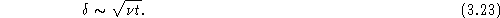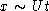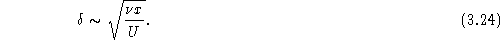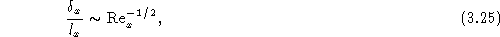Next: Skin friction Up: Viscosity effects at high Previous: Viscosity effects at high

## Boundary layers

One of the simplest flow configurations which illustrates the boundary layer concept is the flow of a fluid parallel to a thin, flat plate; the geometry is shown in Fig. 3.3. See also Tritton, page 102.Figure 3.3: Geometry for viscous flow past a thin plate.

If the fluid were nonviscous, the streamlines would be parallel to the plate and nothing very interesting happens. For a viscous fluid, however, we must apply the no-slip boundary condition on the surface of the plate. The thickness of the boundary layer, which will be denoted by, is the distance required for the velocity profile to approach its free stream value. Recalling that the viscosity is a measure of the diffusion of velocity (or vorticity), the thickness of the boundary layer after a time t is approximately given byNow in a time t an element of fluid which begins at the leading edge of the plate will have moved a distance, so that the boundary layer thickness a distance x from the leading edge isTherefore, the boundary layer thickness at the trailing edge of the plate, measured relative to the length of the plate itself, iswhere . We see that the boundary layer thickness decreases with increasing Reynolds number (for an assumed laminar flow).

Vittorio Celli
Sun Sep 28 22:13:11 EDT 1997